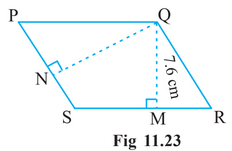# Q. 5.     is a parallelogram (Fig 11.23). is the height from to and is the height from to . If and              Find:(a) the area of the parallelogram

R Riya

We know that

Area of parallelogram

Here,

Base of parallelogram = 12 cm

and

Height of parallelogram = 7.6 cm

Therefore, area of parallelogram is

Exams
Articles
Questions